# YOLO V3 Trained onOpen Images Data

Contributed by: Julian W. Francis

Detect and localize objects in an image

YOLO (You Only Look Once) Version 3 is an object detection model published in April 2018. It is a single-stage architecture that goes straight from image pixels to bounding box coordinates and class probabilities. Compared to previous versions of YOLO, it performs bounding box regression and classification at three different scales and uses three anchor boxes instead of two. Additionally, residual blocks and skip connections are added and L2 losses for object confidence and class predictions are now replaced by cross-entropy error terms.

Number of layers: 349 | Parameter count: 65,252,682 | Trained size: 263 MB |

## Training Set Information

• Open Images, a dataset for image recognition, segmentation and captioning, consisting a total of 16 million bounding boxes for 600 object classes on 1.9 million images.

## Performance

• This model achieves an mAP-50 of 51.5% and an AP50 of 57.9% on the MS-COCO 2014 test set.

## Examples

### Resource retrieval

Get the pre-trained net:

 In:=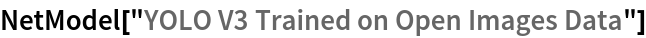Out=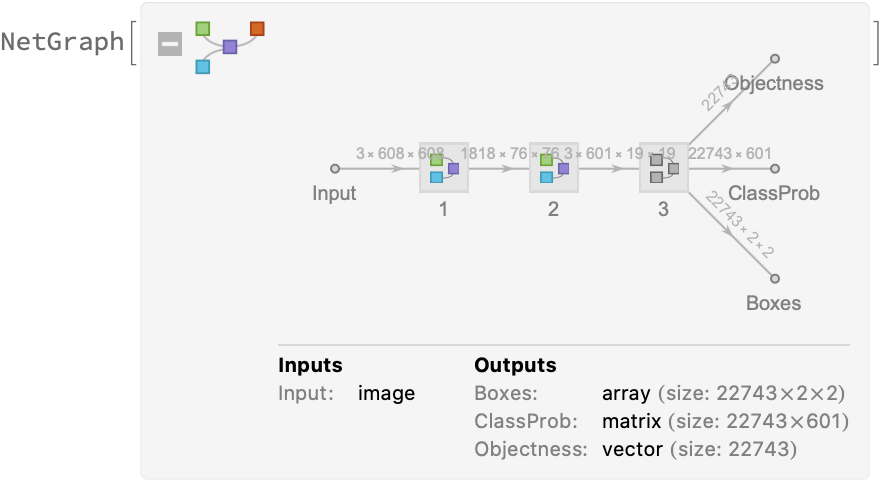### Label list

Define the label list for this model. Integers in the model’s output correspond to elements in the label list:

 In:=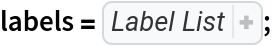### Evaluation function

Write an evaluation function to scale the result to the input image size and suppress the least probable detections:

 In:=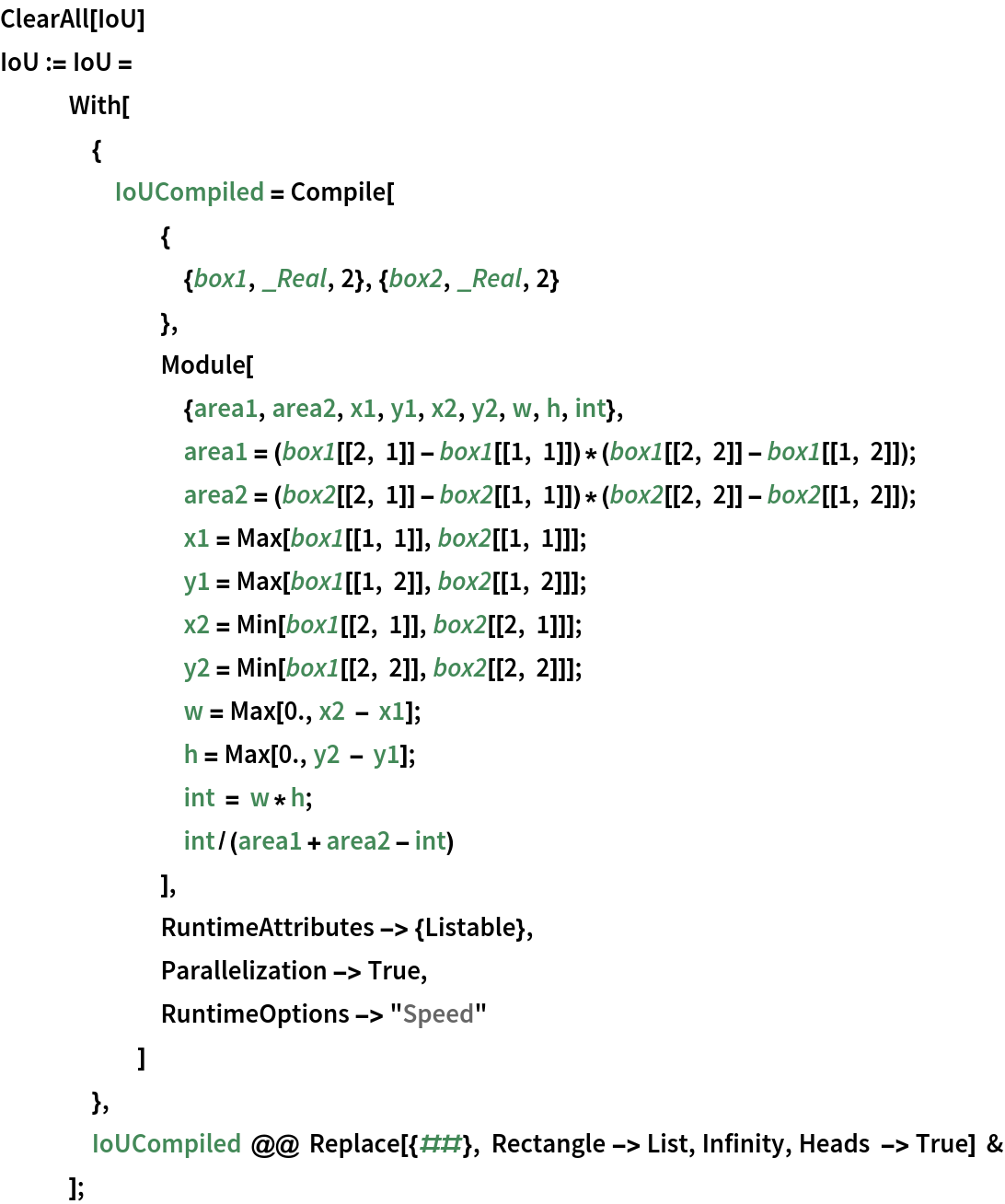In:=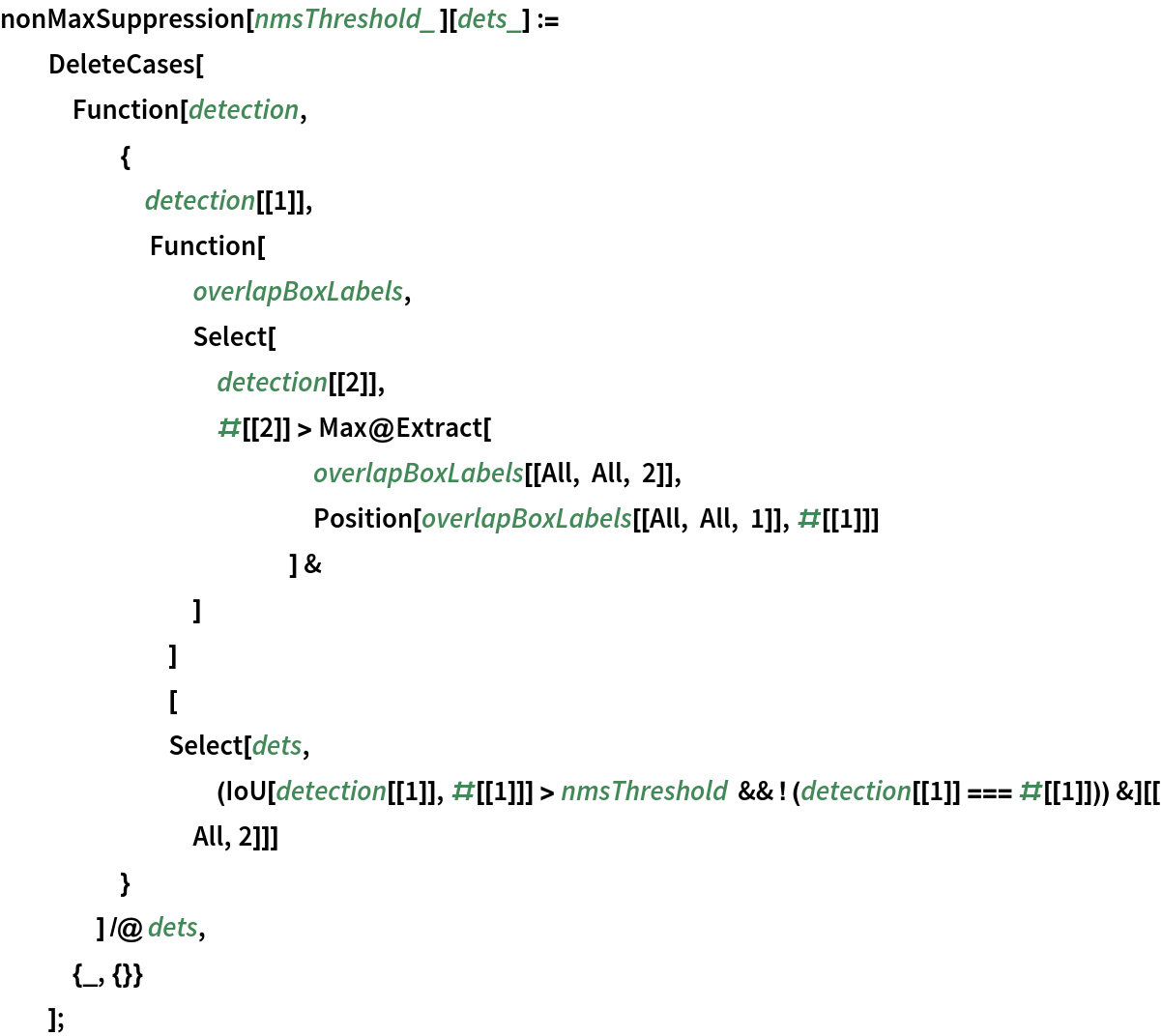In:=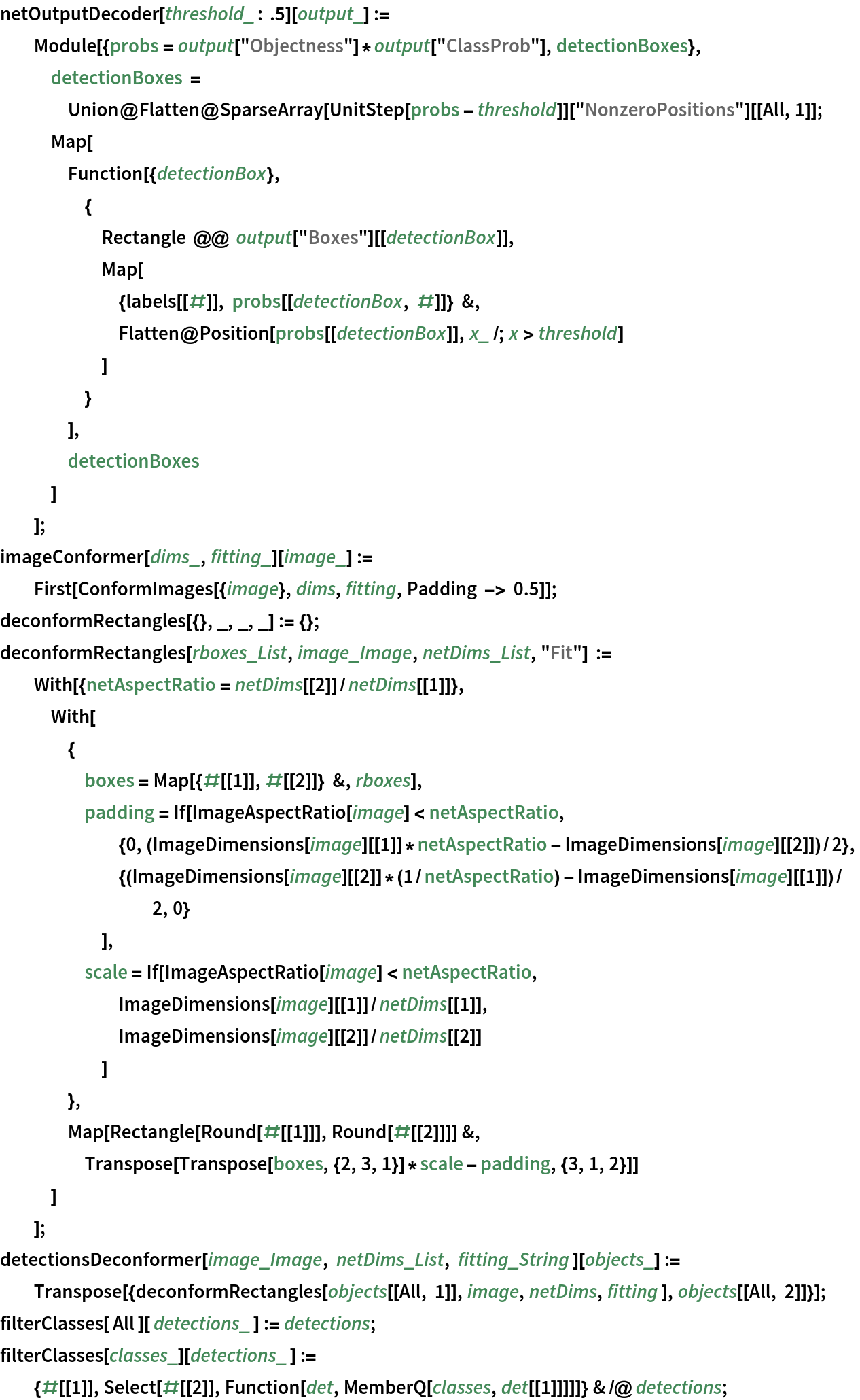In:=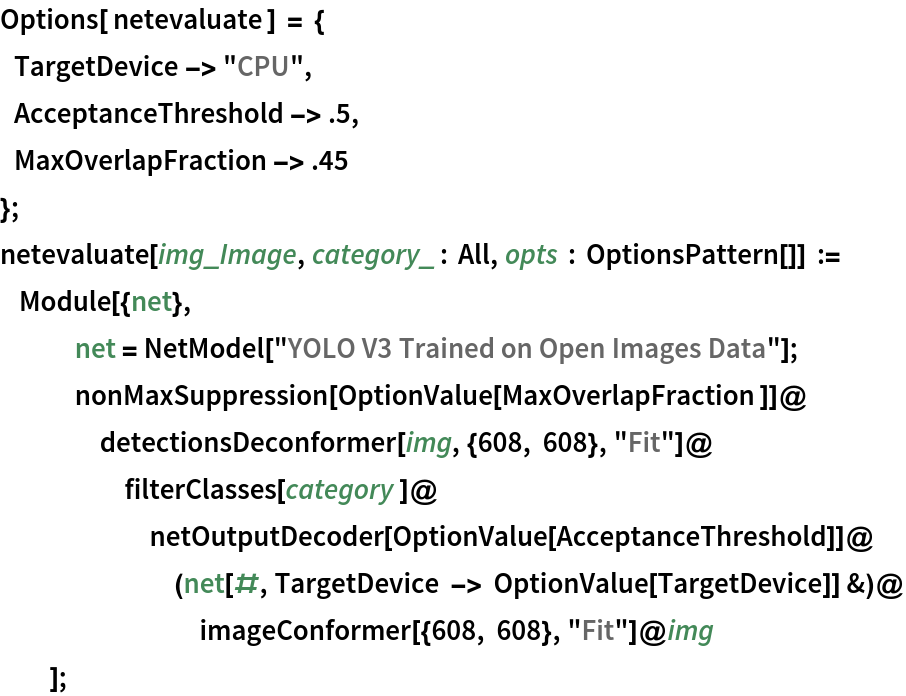### Basic usage

Obtain the detected bounding boxes with their corresponding classes and confidences for a given image:

 In:=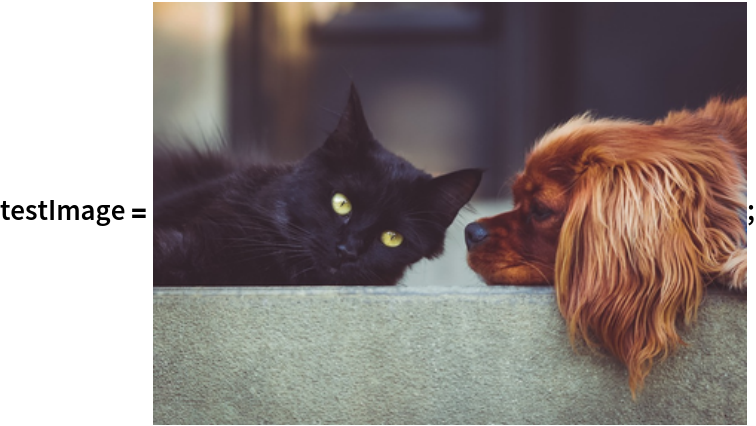In:=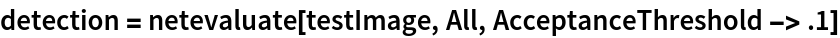Out=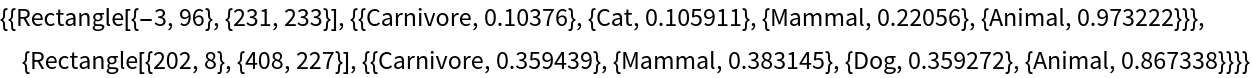Inspect which classes are detected:

 In:=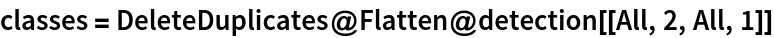Out=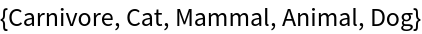Visualize the detection:

 In:=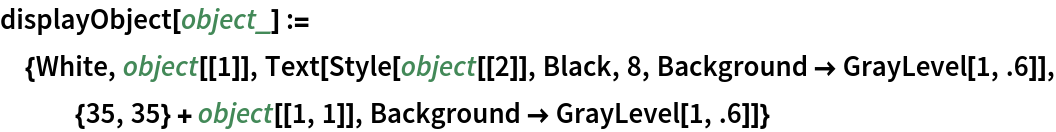In:=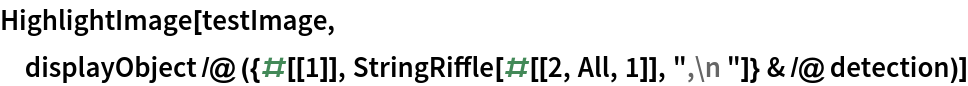Out=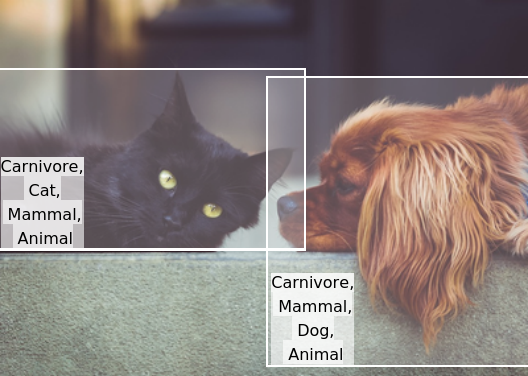### Network result

The network computes 22,743 bounding boxes, the probability of having an object in each box and the conditioned probability that the object is of any given class:

 In:=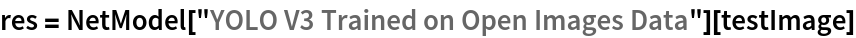Out=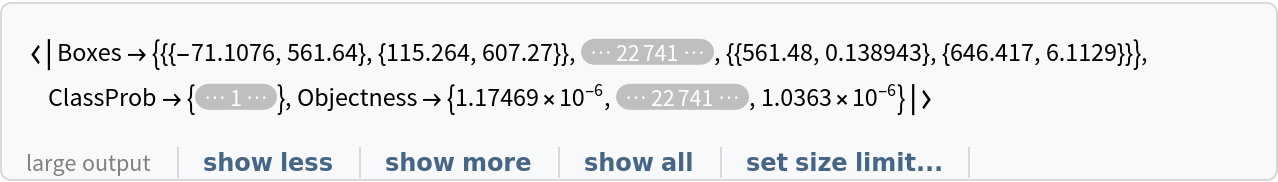In:=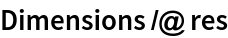Out=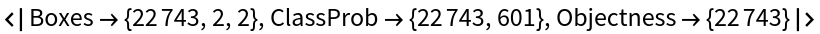Visualize all the boxes predicted by the net scaled by their “objectness” measures:

 In:=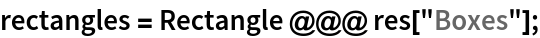In:=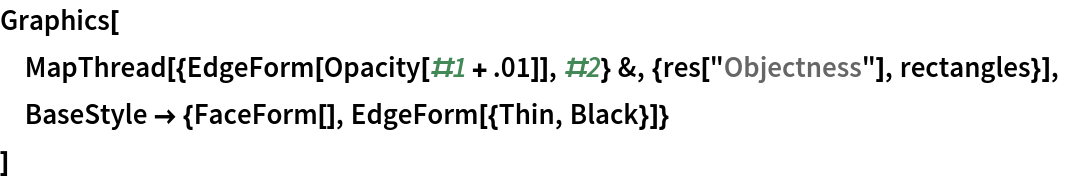Out=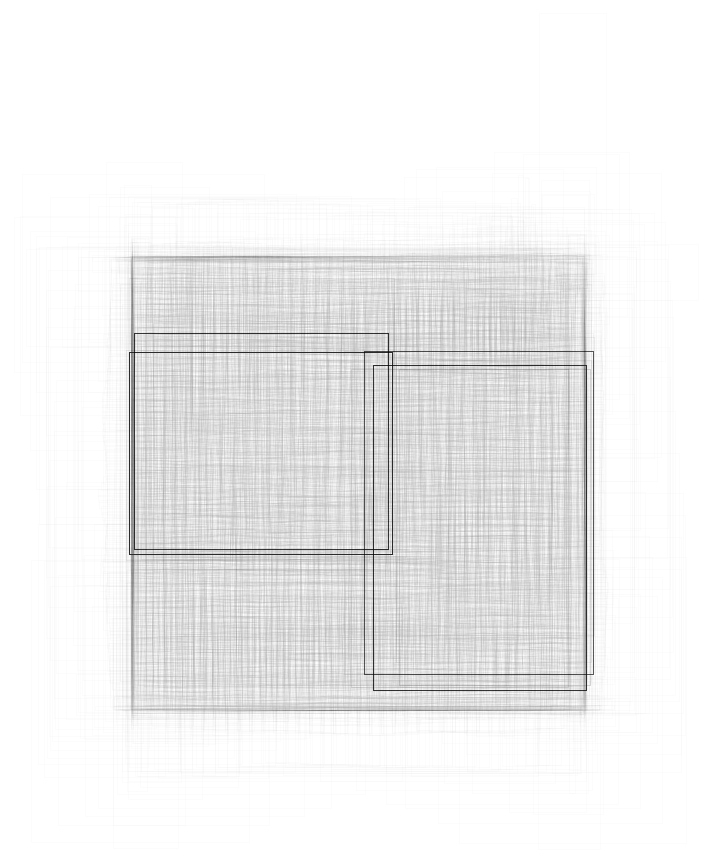Visualize all the boxes scaled by the probability that they contain an animal:

 In:=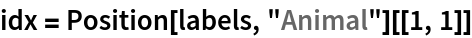Out=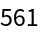In:=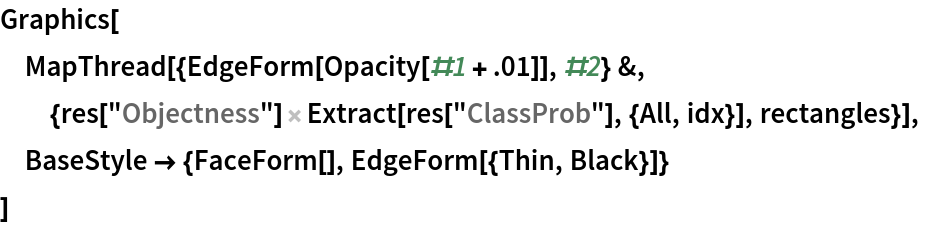Out=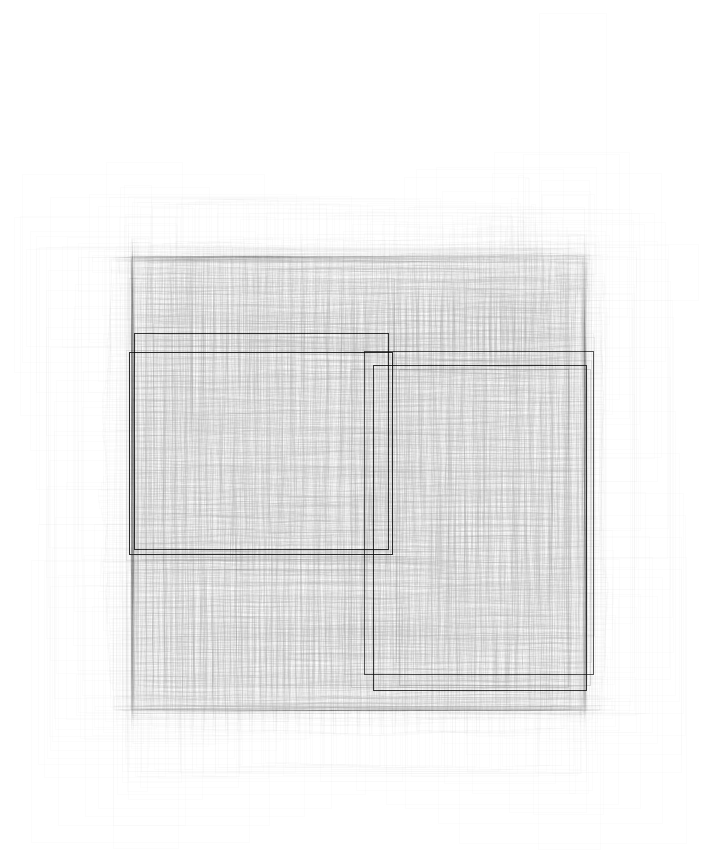Superimpose the animal prediction on top of the scaled input received by the net:

 In:=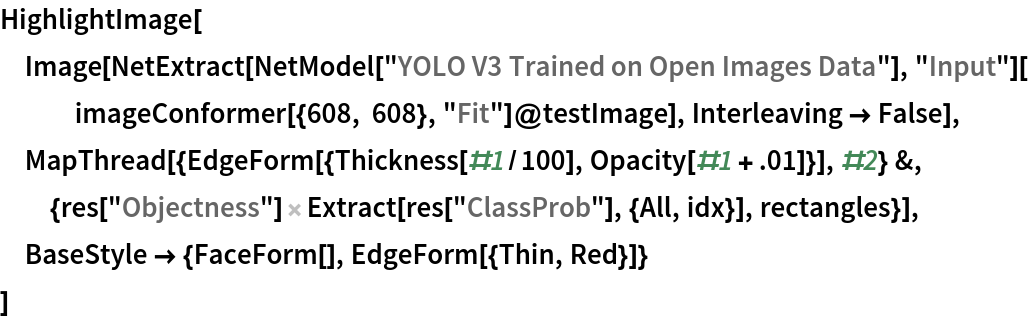Out=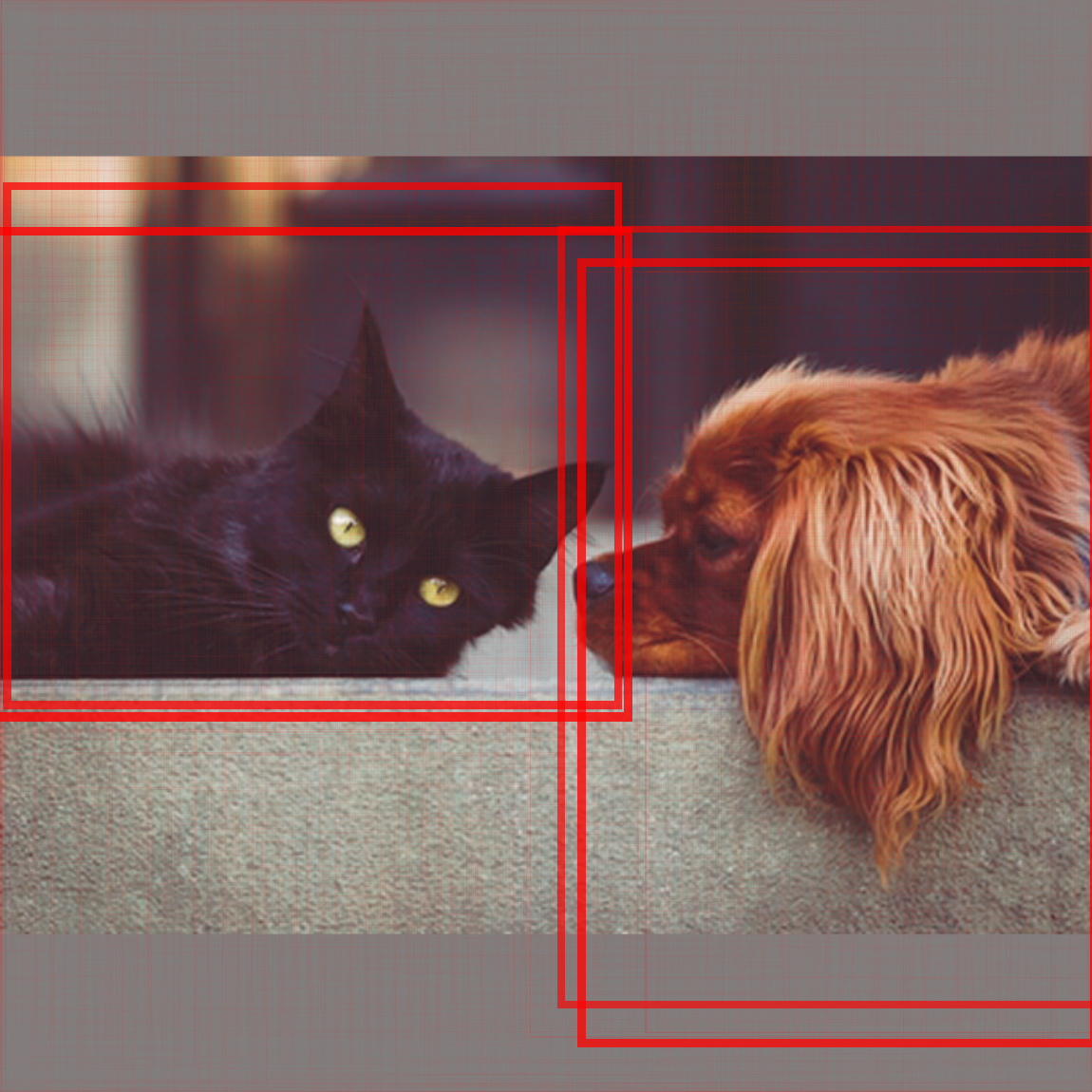### Class filtering

Obtain a test image:

 In:=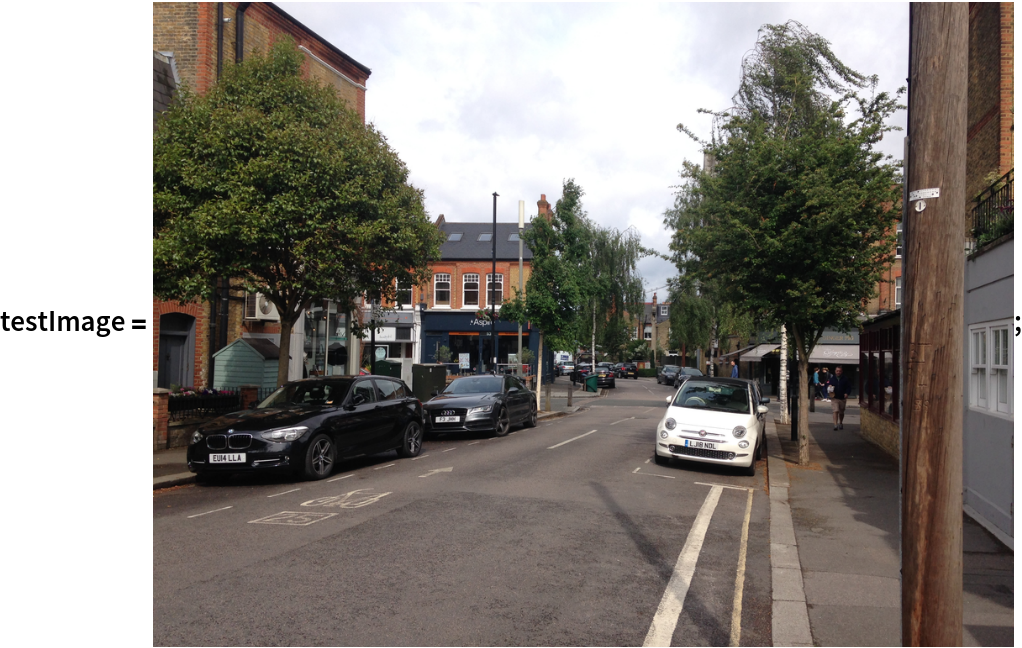Obtain bounding boxes for the specified classes only (“Vehicle registration plate” and “Window”):

 In:=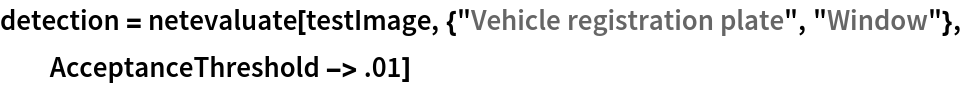Out=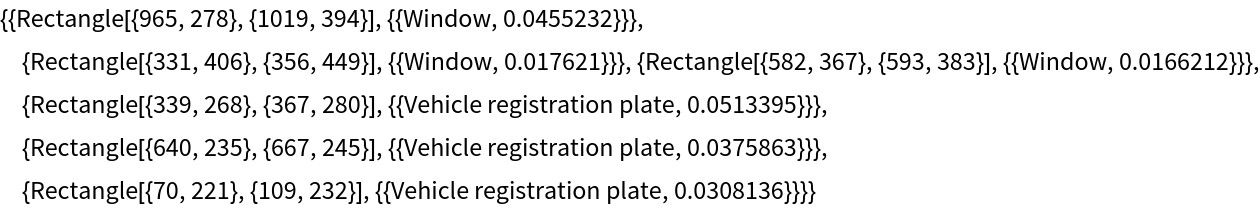Visualize the detection:

 In:=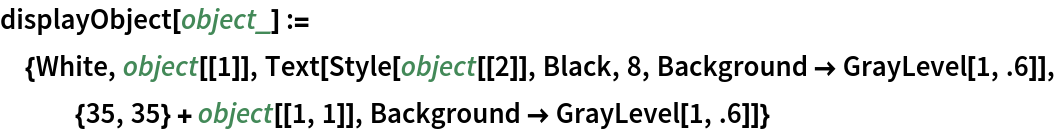In:=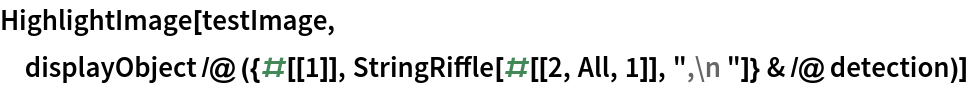Out=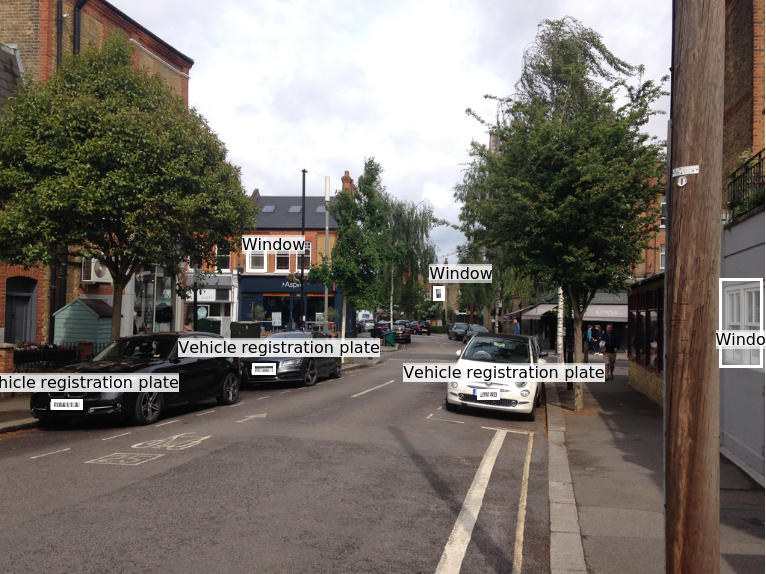### Net information

Inspect the number of parameters of all arrays in the net:

 In:=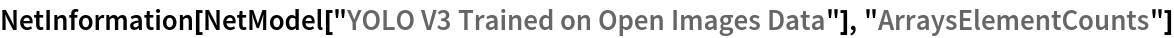Out=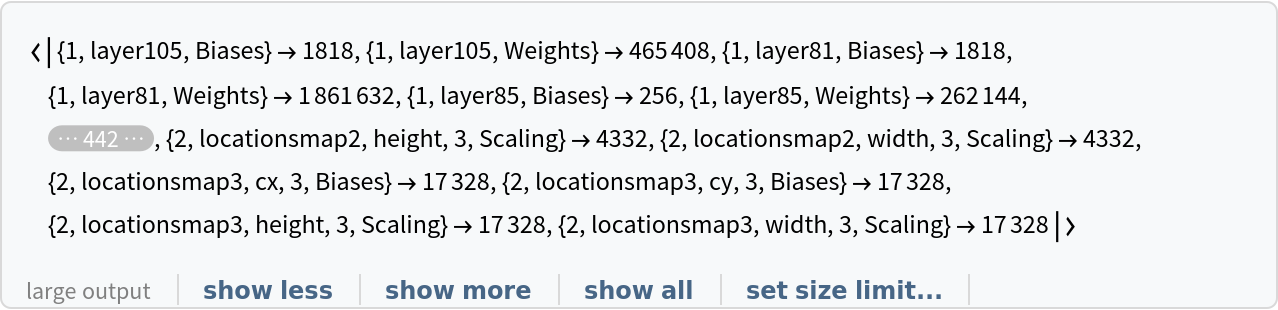Obtain the total number of parameters:

 In:=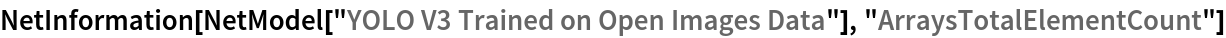Out=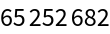Obtain the layer type counts:

 In:=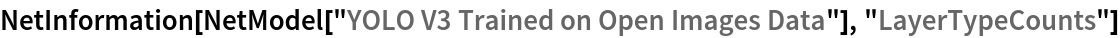Out=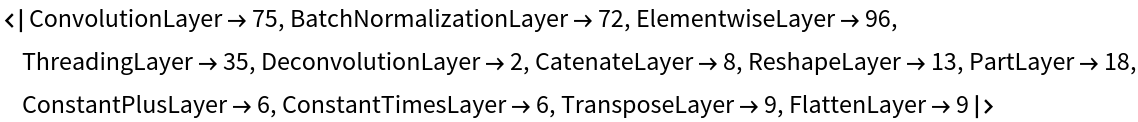Display the summary graphic:

 In:=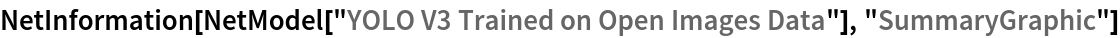Out=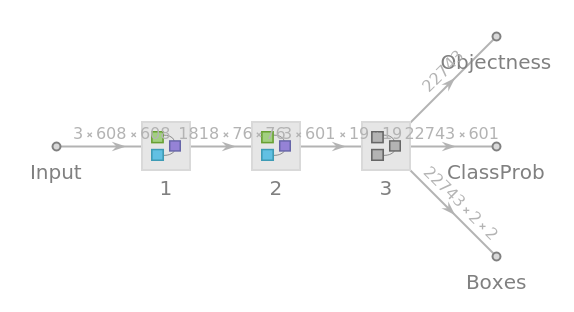### Export to MXNet

Export the net into a format that can be opened in MXNet:

 In:=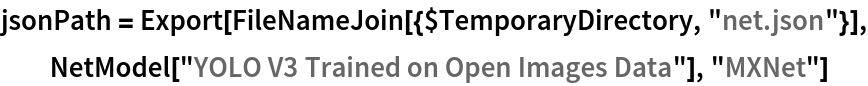Out=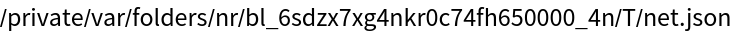Export also creates a net.params file containing parameters:

 In:=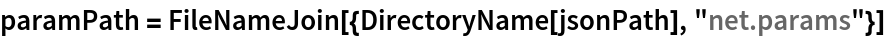Out=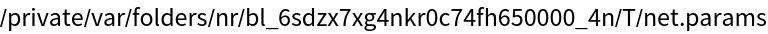Get the size of the parameter file:

 In:=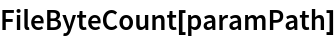Out=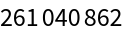The size is similar to the byte count of the resource object:

 In:=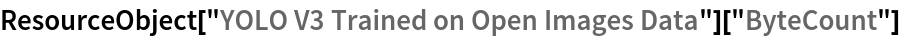Out=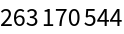## Requirements

Wolfram Language 12.0 (April 2019) or above